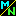Navigation Panel:(These buttons explained below)# What Does "Existence" Mean in Mathematics?

Understanding what existence means in mathematics is the key to understanding what it means for concepts like "infinity" or "imaginary numbers" to exist--something that puzzles a lot of people when they first encounter these weird ideas!

Mathematical objects do not exist in the same sense that a physical object exists; nobody has ever bumped their elbow on a number, for instance.

Instead, mathematical objects are abstract concepts (often abstracted from a real world situation, by isolating just the part of the situation that is relevant for a particular discussion).

When we ask whether or not a mathematical object exists, we must have in mind an appropriate context: a particular, precisely defined collection of concepts. Then we ask, "among these concepts, is there one which matches the object we are looking for?" If so, we say that the object exists; if not, it doesn't exist.

For example, the natural numbers (that is, the numbers 1, 2, 3, 4, and so on) are the concepts obtained by abstracting the property of "size" from collections of objects. The number 2 is the abstract concept that expresses what the following collections of objects have in common: the eyes on a person's face, the occurrences of the letter "b" in the word "bib", the wheels on a bicycle, and so on.

If we were to ask "does there exist a natural number between 1 and 2", we mean, "among the collection of natural numbers, is there one (say x) such that 1 < x < 2?" The answer to this question is "No". You cannot, for example, go to the beach and pick up more than one pebble but fewer than two pebbles.

If we were to ask "does there exist a number between 1 and 2", the answer would depend on what we meant by the word number.

If we were using the word "number" to mean "natural number" (that is, measurement of the size of a set), then the answer would be "No; no such number exists."

However, there are other contexts in which the answer might be "Yes". For example, we might be in a context where number is meant to refer, not to a natural number, but to a rational number: that is, a fraction.

Rational numbers are something quite different from natural numbers: instead of being measurements of sizes of sets, they are ratios of the sizes of two sets. For example, the fraction 3/4 is expressing the ratio "3 to 4".

In this context, where number refers to a ratio not to the size of a single set, and where "2" and "1" really mean the fractions "2/1" and "3/1" respectively, then the answer to our question is "yes": there does exist a number between 1 and 2, for instance the fraction 3/2.

This illustrates that, before one can say whether a concept exists or not, we have to be quite clear about the context in which the question is being asked.

If you are still puzzled by this, you might want to read more (in a discussion of whether or not "imaginary numbers" exist) about how there are many quite different meanings for the word "number", and how whether or not a concept exists can depend on the meaning you have in mind.

Navigation Panel: